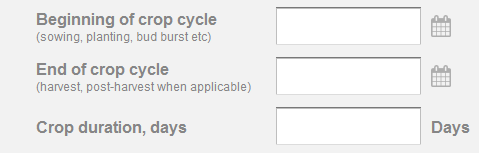Calculate days from date inputs

#1

Hi
I’m trying to calculate days,
I have 2 date inputs:
1- Beginning of crop cycle (date)
2- End of crop cycle (date)
3- a field that needs to sum days total
thanks!!#2

@ness

I guess you only need to get a hint of the implementation, so i come up with this simplified version.

in this example, the assumptions are:

1. date format: MM/DD/YYYY
2. semi-close range of the date [start, end) - do not include the end date

CalculateDuration.rp (47.2 KB)

#3

Hi Ness

This may not quite be the answer you’re looking for, but, perhaps by clicking on calendar 1 “a date” could appear in the first box, clicking on calendar 2, would put another date in the second and show the number of days inbetween? Everything fixed and precalculated just to show the logic?

Afterall, what you’re trying to achieve is quite complex (I just spent 30 minutes trying to come up with something and didn’t get anywhere, must get back to work now)…

Best
Dorota

#4

Hi!

Axure has a number of functions that allow doing math with dates. One such functions converts the provided date to the number of milliseconds that have transpired since an arbitrary date in the 70s.

 Use revised solution below rather than this one.

There are 1000 milliseconds in a second, so to convert this millesecond number to days, you’d divide it by 1000, then by 60 (seconds), then by 60 (minutes) then by 24 (hours). So to get the number of days that have transpired, you’d use this, assuming LV_start is a local variable referring to a field containing a date:

[[ Date.parse(LV_start)/1000/60/60/24 ]]

(The surrounding double-braces tell Axure that this is an expression that it needs to evaluate, as opposed to text that you want to display as is.)

So to get the number of days between a start date and end date (inclusive of the start date):

[[ (Date.parse(LV_end)/1000/60/60/24 - Date.parse(LV_start)/1000/60/60/24).toFixed() + 1 ]]

Example file: calculate_days_between_two_dates.rp (51.2 KB)

I forgot to consider that a day is shorter than 24 hours, due to leap year, etc. (I was wondering why I had to round it.)

Instead, use 86400000: the number of milliseconds in a day, according to the javascript date functions.

[[ Date.parse(LV_end)/86400000 - Date.parse(LV_start)/86400000 + 1 ]]

LV_end and LV_start are local variables with the two dates, Date.parse() converts each date to milliseconds since 1970, which is converted to number of days since 1970 by the division. The subtraction gives the difference in days. The 1 is added to so the span goes from the morning of the first day to the night of the last day, rather than just morning to morning. So Jan 1 through Jan 31 gives us 31 days instead of 30. (This may or may not be desired.)

Preview

Updated file: calculate_days_between_two_dates.rp (51.7 KB)